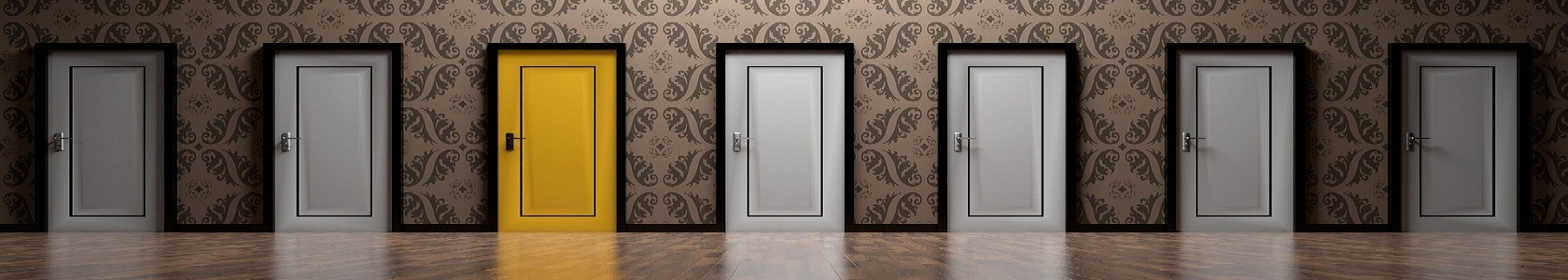# Nursing quiz

##### Nursing Research Quiz - 131 2 3 4 5 6 7 8 9 10 11 12 13 14 15 16 17 18 19 20 21 22 23 24 25 26 27 28 29 30

#### Nursing Research Quiz - 13

##### biostatistics

1. In a drug trial, one group showed 40% response and the group with the test drug showed 60% response. The two results can be statistically compared for significance by:

A. Chi-square test

B. Paired test

C. t-test with different measures

D. Fischer test

2. In a group of 100m children, the weight of child is 15 kg. The standard error is 1.5 kg. Which of the following is true?

A. 95% of all children weight between 12 and 18 kg

B. 95% of all children weight between 13.5 and 16.5 kg

C. 99% of all children weight between 12 and 18 kg

D. 99% of all children weight between 12 and 18 kg

3. The following statistics is used to measure the linear association between two characteristics in the same individuals:

A. Coefficient of variation

B. Coefficient of correlation

C. Chi-square

D. Standard error

4. Which of the following test of significance can be used to compare unrelated variables when values are all binary?

A. t-test

B. Chi-square test

C. Proportion test

D. Correlation test

E. Regression test

5. What is true about Chi-square test?

A. Expected and observed values are always equal

B. Mutually exclusive

C. Mutually non-exclusive

D. Indicates median

6. In Chi-square test degree of freedom 1, Chi-squire value= 6.7, then P-value will be:

A. > 0.5

B. <. 0.1

C. < 1 .0

D. < 0. 05

7. Regarding paired t-test true is:

A. Hypertension in a person before and after treatment can be studied

B. Continuous variables in a single sample

C. Different variables in a single sample

D. Unrelated samples can be compared

8. Complete correlation between height and weight is best given by:

A. -1

B. +1

C. 0

D. Infinity

9. Which of the following is a pre-requisite for the Chi-square test compare?

A. Measures should be in continuous scale

B. Both samples need not be mutually exclusive

C. Both samples should be mutually exclusive

D.. Samples should follow normal distribution

10. The Chi-square test is used to measure the degree of:

A. Causal relationship between exposure and effect

B. Correlation between two variables

C. Association between two variables

D. Agreement between two observations